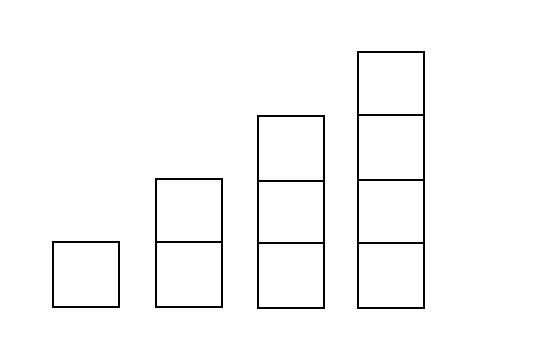# 2021 年东华大学金马程序设计联赛

J. A Special Game
PDF 题面可用

“Largest Rectangle in Histogram” problem is a classic problem. You are given an array of integers where each element represents the height of a bar in a histogram. A histogram is a graphical display of data using bars of different heights. The bars are placed in the exact same sequence as given in the array, and each of them has width 1. You need to find the area of the largest rectangle in the histogram.

But now Alice and Bob want to play a game on a histogram.They take alternating turns, and Alice is moving first.

Initially all cells are empty.During each turn, the player chooses three numbers:
$i,j,k(1\leq i\leq n,1\leq j\leq a_i-k,0\leq k\leq 3)$, then paints the following cells:$(i,j),(i,j+1),(i,j+2)\cdots(i,j+k)$. Remeber these cells must be empty area that has not been painted before.On the next turn another player does the same, then the first one and so on. The player with no cells to paint on his turn loses.

You have to determine who wins if they both play optimally.

### 输入格式

There are multiple test cases in this problem.($T\leq 100$)

In each test case,the first line contains one integer n denoting the width of histogram($1\leq n\leq 10^4$).

The second line contains n space-separated positive integers $a_i$ denoting the height of the i-th rectangle($1\leq a_i\leq 10^6$).

### 输出格式

For each test case print Emo:? if Alice can win and lets see who is real evil genius if Bob can win.

### 样例

Input
2
1 1
1
4
1
5
1
9
3
15 16 17
3
1000000 1 2

Output
lets see who is real evil genius
Emo:?
Emo:?
Emo:?
Emo:?
lets see who is real evil genius


NaN